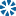##### (+91) 9693466662, 7302623597
Mon - Sat 08:00 AM to 07:00 PM Sun 9:30 AM to 5:30 PM

## Our Blog

• 16-Sep-2021
###### SUCCESSIVE PERCENTAGE PART-03

For successive increase/decrease in percentages, for successive years/times, the following formula will come handy: Let the successive increase in percentages be a%a% and b%b%. In that case, the total increase will be (a+b+ab100)%(a+b+ab100)% Lets try an example: If the increase is 10% and 20 %, the successive increase will be (10+20+20∗10100)=32%(10+20+20∗10100)=32%. If there`s an increase and a decrease, in that case, the decrease will be considered a negative value. Lets try an example. If there`s an increase of 20% and then a decrease of 10%, the successive percentage will be (20+(−10)+20∗(−10)100)=20−10−2=8%(20+(−10)+20∗(−10)100)=20−10−2=8% increase. In case of discounts, the value of discount percentages will be considered negative. Lets try an example: If Kouton`s give 50% + 50% off on independence day, what is the final discount given by Koutons The discount percentage will be (−50+(−50)+(−50)∗(−50)100)=−100+25=75%(−50+(−50)+(−50)∗(−50)100)=−100+25=75% discount. This method is useful when the percentage increase / decrease if for lot many times. Once this method is mastered, successive percentage calculation will be a cakewalk and would relieve you of the pain of picking up pencil and scribing on paper.. :-) Hope the methods helps you all.

• 10-Mar-2021
###### Successive Percentage Change (Questions)-02

One percent is a hundredth. As a percent sign, we usually use %, but sometimes it is denoted by pct. It means that 5 percent is the same as 5%, 5 pct, 0.05, 5/100, or five-hundredths. It is as simple as that, and this percentage calculator is a tool dedicated to working with decimal fractions and percentages

• 09-Mar-2021
###### Successive Percentage Change (Questions)-1

Percentage Formula Percentage formula is used to find the amount or share of something in terms of 100. In its simplest form, percent means per hundred. To express a number between zero and one, percentage formula is used. It is defined as a number represented as a fraction of 100. It is denoted by the symbol %, and is majorly used to compare and find out ratios. Formula to Calculate Percentage The Percentage Formula is given as, Percentage = (Value ⁄ Total Value)

• 18-Feb-2020
###### UGC NET for Commerce Preparation Tips

UGC NET is considered as one of the toughest exams to clear as compared to the seats available,huge numbers of candidates appear for this exam every year. The aspiring candidates may follow a few helpful tips provided below in order to excel in the UGC NET 2020 exam.

• 26-Jan-2020

CTET 2020 examination scheduled has been announced. Application Form (24th January to 24th February 2020), Eligibility Criteria, Syllabus, Exam Pattern and Preparation Tips.

• 26-Jan-2020
###### Best SSC CHSL Coaching in Ranchi

SSC Conducts combined Higher Secondary level examinations for 10+2 passed students for Postal Assistants/Sorting Assistants, Data Entry Operators, Lower Divisional Clerks and Court Clerk posts. These examinations are conducted almost every year by SSC for the recruitment of clerical & data entry staff in the departments of different ministries.